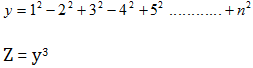# Some Java program part 6

1. Write a Java program that would input three numbers from the user and print sum, then the first number, then the 2nd number followed by 3rd number. If user enters 10, 20, 30. then output should be 60, 10, 20, 30.
```import java.util.Scanner;
public class Lab8q4{
public static void main ( String [] args ){
int [] number = new int ;
int c = 0, sum=0;
Scanner input = new Scanner ( System.in );
System.out.println(&quot;Enter 3 numbers&quot;);
while(c&lt;3){
number  = input.nextInt();
sum = sum + number ;
++c;
}
System.out.println(&quot;SUM : &quot;+sum);
c=0;
while(c&lt;3){
System.out.println(number);
++c;
}
}
}
```
1. Write a Java program that would input ten numbers from the user and print the ten numbers in reverse order. If user enters 20, 10, 30, 15. Then output should be 15, 30, 10, 20.
```import java.util.Scanner;
public class Lab8q5{
public static void main ( String [] args ){
int [] number = new int ;
int c = 0, sum=0;
Scanner input = new Scanner ( System.in );
System.out.println(&quot;Enter 3 numbers&quot;);
while(c&lt;3){
number  = input.nextInt();
sum = sum + number ;
++c;
}
c=2;
while(c&gt;=0){
System.out.println(number);
--c;
}
}
}
```

# Some Java programs part-5

21. Write a program that will calculate the value of Z  if the expression of y is as follows (n is the input):# Some Java programs part 4

16. Write a program that will read 10 numbers from the user, and then print the first number, the sum of the first 2 numbers, first 3 numbers, and so on up to the sum of 20 numbers.

```/*Time : 5:00pm
* Author: Anik das*/
import java.util.Scanner;
public class Faraz16{
public static void main ( String [] args ){
int c=1, sum=0, number;
Scanner input = new Scanner ( System.in );
while (c&lt;=10){
System.out.println(&quot;Enter Number #&quot;+c);
number = input.nextInt();
sum=sum+number;
System.out.println(&quot;Sum Of 1st &quot;+c+&quot; Number(s) is : &quot;+sum);
c++;
}
}
}
```

# A little about array in Java

I have seen many of my friends to have trouble with the problems in array. So, this time I have selected to write with array…don’t know how much effective it would be to you guys…

First of all, what is array? Normally we can say that array is a static memory allocation. For example we can think an array as a number of conjoined boxes where we can store our desired values of same data type. Now the question may arise that we can use normal variables to store data, why use array? The answer is we should use array because it saves our time and minimizes the memory usage of the machine. For example: suppose that you have to store marks of 5000 students of your university. Now if you want to store those marks using normal variables firstly you have to select 5000 variables (which would be horrible!). Then, you will need to declare each variable (int a, b, c…up to 5000 variables). Than you have to initialize each variable too, haven’t you? In this case if you use array your task will become easier. All you have to do is, firstly you have to declare an array. Secondly, you have to initialize that the array will be consist of 5000 indexes it means the array will consist of 5000 conjoined boxes where the marks will be stored.

# Before you start java programming

There are some prerequisites to start java programming. Make sure have these following things.

1. Software to write JAVA program
2. Java runtime 1.5 or above

For the first one I would recommend Dr.Java as I am much familiar  with it because it’s used in my University. You can find out Dr.Java in Dr.java.org . You cannot run Dr.Java if you do not have java runtime 1.5 or above installed in you machine. To install java in your computer follow the next step.